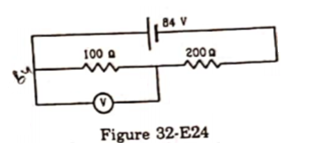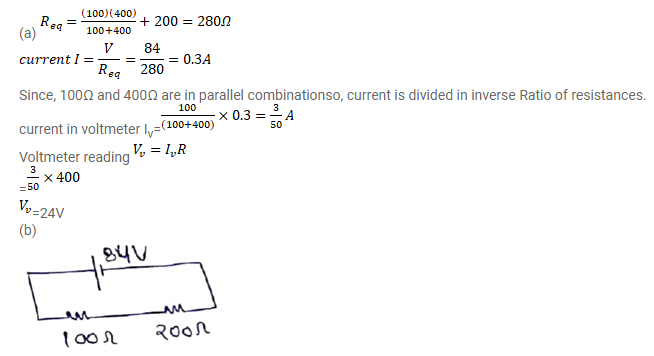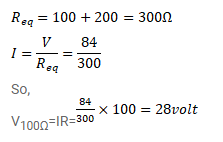# A voltmeter of resistance 400Ω is used to measure

Question:

A voltmeter of resistance $400 \Omega$ is used to measure the potential difference across the $100 \Omega$ resistor in the circuit shown in figure (32-E24).

(a) What will be the reading of voltmeter?

(b) What was the potential difference across $100 \Omega$ before the voltmeter was connected?Solution: